We think you are located in Nigeria. Is this correct?

Practise now to improve your marks

You can do it! Let us help you to study smarter to achieve your goals. Siyavula Practice guides you at your own pace when you do questions online.

Chapter 1: Numbers big and small

Last year you learnt how to write and read numbers up to one million. You also counted in millions. In this chapter, you will revise some of last yearâ€™s work. You will also learn to read, write and count in even larger numbers. To help you to do this, you will first learn more about our main system of counting.

1.1 The base ten number system

The most common number system used for everyday counting is the base ten number system. It is also called the decimal number system. The number 10 is the base of this number system.

When we count using the base ten number system, we actually put objects in groups of ten. For example, count these stars:

There are 12 stars. The number 12 means we have 1 group of ten stars and 2 stars left over.

In this system, we use the numbers 0; 1; 2; 3; 4; 5; 6; 7; 8; 9 to make any other number, no matter how big or how small it is. When the numbers 0; 1; 2; 3; 4; 5; 6; 7; 8; 9 are used as part of another number, we often call them digits.

base ten number system The base ten number system is a number system that counts in groups of 10, and uses uses the digits 0; 1; 2; 3; 4; 5; 6; 7; 8; 9 to represent any other number. It is also called the decimal number system.

digits Digits are the numbers 0; 1; 2; 3; 4; 5; 6; 7; 8; 9 that are used to form other numbers.

In the base ten number system, we go to a new group each time we reach the number 10. The numbers 1 to 9 are called units. Zero is used as a placeholder. The number after 9 is 10, which means that we have 1 group of ten and nothing left over. The zero shows that there are no units in the number 10.

We may count in tens until we reach 9 groups of 10: 10; 20; 30; 40; 50; 60; 70; 80; 90. When we add another group of ten, we have 100. The number 100 means that we have 1 group of 100, zero groups of ten and zero units.

Similarly, we may count in hundreds until we reach 9 groups of 100: 100; 200; 300; 400; 500; 600; 700; 800; 900. When we add another group of 100, we have 1,000. The number 1,000 means that we have 1 thousand, zero hundreds, zero tens and zero units.

When we count in thousands, 1,000; 2,000; 3,000; 4,000; 5,000; 6,000; 7,000; 8,000; 9,000, we eventually reach 10 groups of 1,000, which is 10,000. This number is called ten thousand.

units Units are the numbers 1; 2; 3; 4; 5; 6; 7; 8; 9 in the base ten number system.

tens Tens are the numbers 10; 20; 30; 40; 50; 60; 70; 80; 90 in the base ten number system.

hundreds Hundreds are the numbers 100; 200; 300; 400; 500; 600; 700; 800; 900 in the base ten number system.

thousands Thousands are the numbers 1,000; 2,000; 3,000; 4,000; 5,000; 6,000; 7,000; 8,000; 9,000 in the base ten number system.

ten thousands Ten thousands are the numbers 10,000; 20,000; 30,000; 40,000; 50,000; 60,000; 70,000; 80,000; 90,000 in the base ten number system.

Grouping digits

You may have noticed that we write a thousand as 1,000 rather than 1000. Similarly, we write ten thousand as 10,000 rather than 10000. We use a comma to group the digits. Grouping digits makes it easier to read large numbers.

Later you will work with decimal fractions. An example of a decimal fraction is 0.25. It is the same as $\frac{1}{4}$. We use a decimal point to separate whole numbers from fractions. In the number 2.5, the decimal point separates the whole number 2 from the fraction 0.5.

Usually, we group only the digits representing whole numbers, that is, the digits to the left of the decimal point. The digits are grouped in threes, starting at the decimal point and going to the left. For example, the number 2516.2 is written as 2,516.2 and not as 251,6.2.

Exercise 1.1: Group the digits in large numbers

Rewrite each of the following numbers with the digits correctly grouped.

1. 25618.231

25,618.231

2. 100985.0021

100,985.0021

3. 201050.11

201,050.11

4. 5555.55

5,555.55

5. 121212.1212

121,212.1212

In some countries, people use spaces instead of commas to group digits. For example, they write the number 504,816 as 504Â 816. In some countries, they also use a decimal comma instead of a decimal point. For example, they write the number 1,500.5 as 1Â 500,5.

1.2 Place value

Place value in numbers larger than zero

Each digit in a number tells you the specific value of that digit. The place value of a digit tells us what the value of that digit is, based on that numberâ€™s position (place) in the number.

place value Place value is the value of a digit according to its position in a number.

Worked example 1.1: Finding the place value of a digit

Give the place value of each digit in the number 52,143.

1. Step 1: Create a table that represents the place values, with one column for each place. Put each digit of the number into the correct position in the table.

2. Step 2: Use the table to help you to write down the place value of each digit.

There are 5 groups of 10,000, so the value of this 5 is $5\times 10,000=50,000$.
There are 2 groups of 1,000, so the value of this 2 is $2\times1,000=2,000$.
There is 1 group of 100, so the value of this 1 is $1\times100=100$.
There are 4 groups of 10, so the value of this 4 is $4\times10=40$.
There are 3 units, so the value of this 3 is $3\times 1=3$.

Exercise 1.2: Find the place value of a number

Use place value tables to determine the place value of the number 6 in each number.

1. 164

The place value is: $6\times10=60$

2. 1,026

The place value is: $6\times1=6$

3. 68,417

The place value is: $6\times10,000=60,000$

4. 13,650

The place value is: $6\times100=600$

5. 26,009

The place value is: $6\times1,000=6,000$

Place value in decimal fractions

A fraction means a part of a whole. One way to write a fraction is to write one whole number on top of another whole number with a dividing line between them. For example, $\frac{2}{10}$ means two parts of a whole that is divided in ten.
The name of the top number is the numerator, and the name of the bottom number is the denominator: $\dfrac{\text{numerator}}{\text{denominator}}$

A decimal fraction is a way to write a fraction using the base ten number system. Instead of writing one number on top of another, we write only the numerator after a decimal point. For example, the decimal fraction 0.2 means the numerator is 2 and the denominator is 10, so we may write it as $\frac{2}{10}$.

decimal fraction A decimal fraction is a fraction in which the digits of the numerator are written after a decimal point.

To write a fraction as a decimal fraction, the denominator has to be in a grouping of 10, so it needs to be 10; 100; 1,000; or 10,000, and so on. This is because decimal fractions use the base ten number system.

You will learn more about fractions in Chapter 4. You will also learn how to change a fraction so that its denominator is in a grouping of 10.

For decimal fractions, the place values we work with are $\frac{1}{10},\space\frac{1}{100},\space \frac{1}{1,000}$ and $\frac{1}{10,000}$. They are called tenths, hundredths, thousandths and ten thousandths.

These place values are represented from biggest to smallest, moving to the right after the decimal point:

The fractions for these place values are written in this way:

• 0.1 means zero units and one $\frac{1}{10}$
• 0.01 means zero units, zero tenths and one $\frac{1}{100}$
• 0.001 means zero units, zero tenths, zero hundredths and one $\frac{1}{1,000}$
• 0.0001 means zero units, zero tenths, zero hundredths, zero thousandths and one $\frac{1}{10,000}$.

As for whole numbers, we move to a new place value each time we reach 10. For example, this block of squares is divided into 100 parts.

One hundredth, which is $\frac{1}{100}$, may be shown like this: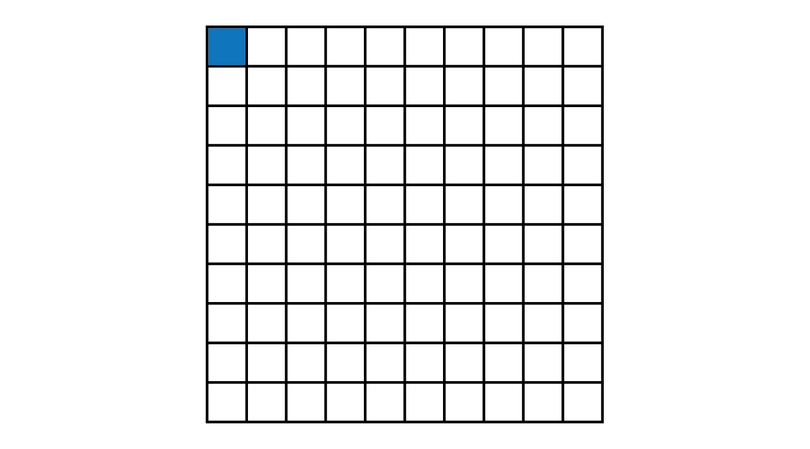Four parts of this block of 100 squares, which is $4\times \frac{1}{100}= \frac{4}{100}$, may be shown like this: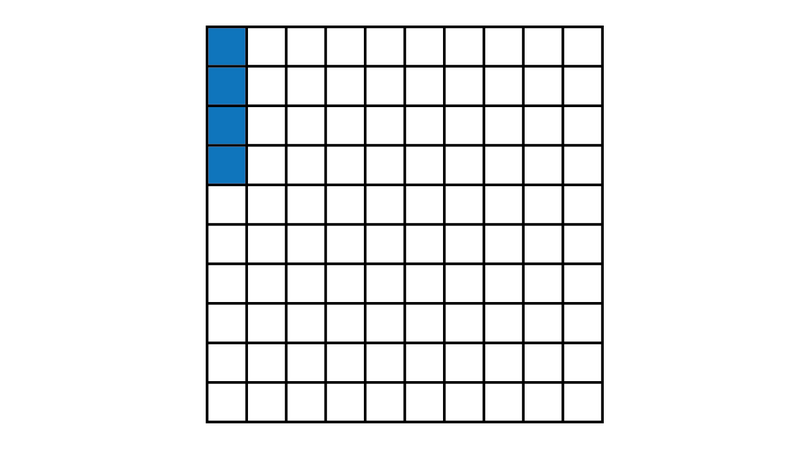Ten parts of $\frac{1}{100}$, in other words, $10\times\frac{1}{100}$, may be shown like this: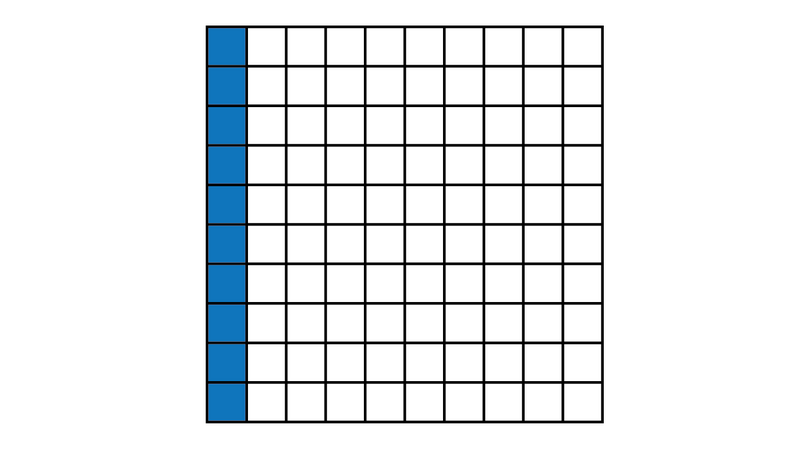There are 10 vertical columns in the block of squares. If 1 column out of 10 is shown in colour, we can write this as $\frac{1}{10}$. Therefore, 10 parts of $\frac{1}{100}$ is the same as $\frac{1}{10}$.

Similarly:

• 10 parts of $\frac{1}{1,000}$ is the same as $\frac{1}{100}$
• 10 parts of $\frac{1}{10,000}$ is the same as $\frac{1}{1,000}$.

tenths Tenths are the decimal fractions 0.1; 0.2; 0.3; 0.4; 0.5; 0.6; 0.7; 0.8; 0.9 in the base ten number system.

hundredths Hundredths are the decimal fractions 0.01; 0.02; 0.03; 0.04; 0.05; 0.06; 0.07; 0.08; 0.09 in the base ten number system.

thousandths Thousandths are the decimal fractions 0.001; 0.002; 0.003; 0.004; 0.005; 0.006; 0.007; 0.008; 0.009 in the base ten number system.

ten thousandths Ten thousandths are the decimal fractions 0.0001; 0.0002; 0.0003; 0.0004; 0.0005; 0.0006; 0.0007; 0.0008; 0.0009 in the base ten number system.

Worked example 1.2: Finding place value in decimal numbers

Write down the place values of all the non-zero digits in the following number: 73,025.048

1. Step 1: Write the number in a place value table. Show the decimal point clearly in your table.

2. Step 2: Multiply each non-zero digit by the value of the column it is in.

$7\times10,000=70,000$
$3\times1,000=3,000$
$2\times10=20$
$5\times1=5$
$4\times 0.01=0.04$
$8\times 0.001=0.008$

As you can see, we do not give the place value of the zeroes in a number, because 0 multiplied by anything equals 0. So the place value of any 0 will always be 0. The zeroes have to be included in a number to show the place values of the other digits. This is why we call 0 a placeholder.

Exercise 1.3: Determine place value in numbers with decimal fractions

Write down the place value of the given digit in each case.

1. Give the values of the digits 5 and 8 in the number 5,023.08

The place values are:
$5\times1,000=5,000$
$8\times 0.01=0.08$

2. Give the values of the digits 1 and 4 in the number 75.1804

The place values are:
$1\times0.1=0.4$
$4\times 0.0001=0.0004$

3. Give the values of the digits 9 and 7 in the number 70,546.009

The place values are:
$7\times10,000=70,000$
$9\times 0.001=0.009$

4. Give the two values of the digit 5 in the number 10,503.54

The two place values are:
$5\times100=500$
$5\times 0.1=0.5$

5. Give the three values of the digit 9 in the number 99,148.319

The three place values are:
$9\times10,000=90,000$
$9\times1,000=9,000$
$9\times 0.001=0.009$

1.3 Powers of ten

In the base ten number system, we can use powers of ten to write big numbers, so that they are easier to read and work with. For example, the number 100 is equal to $10\times 10$. This can be written as $10^2$. Here the number 2 is the power or index of 10. It tells us how many times 10 must be multiplied by itself. A negative power means that we are dealing with a fraction. For example, $10^{-2}$ means $\dfrac{1}{10\times10}$.

You will learn more about writing numbers in index form in Chapter 2.

The table lists the powers of ten for numbers you have already worked with.

This table lists the fractions you have already come across.

1.4 How to add in groups of ten using place value

In the base ten number system, we can have a maximum of 9 digits for one place value. For example, the number 90 means 9 groups of 10, so we have a 9 in the tens place value. We may write it as 9Â Ã—Â 10Â =Â 90. If we add another group of 10, we have 10Â Ã—Â 10Â =Â 100. This means we have 1 group of 100, so we have a 1 in the hundreds place value. Ten groups of 10 is named one hundred.

Similarly, the number 900 means 9 groups of 100. We may write it as 9Â Ã—Â 100Â =Â 900. So there is a 9 in the hundreds place value. If we add another group of 100, we have 10Â Ã—Â 100Â =Â 1,000. So we will then have a 1 in the thousands place value. Ten groups of 100 is named one thousand.

Here are the names of some large numbers made up of groups of 10.

Beyond the digit 9

Whenever we reach the digit 9 in any column of a place value table, and then want to add one more group:

• the digit 9 becomes 0
• the digit in the column to the left must increase by 1.

Worked example 1.3: Dealing with the digit 9

Add 10,000 to the number 591,432.

1. Step 1: Write the given number in a place value table. Find the column in which the digit will have to change.

If 10,000 is added, the digit in the column labelled â€œtthâ€ will change.

2. Step 2: Change the 9 to 0. In the column to the left, increase the digit by 1.

3. Step 3: Write down the answer with the digits grouped in threes.

Exercise 1.4: Do addition with numbers that have the digit 9

1. Add 1,000 to the number 89,015.

Change the 9 to 0 in the â€œthâ€ column, and add 1 to the â€œtthâ€ column.

2. Add 100 to the number 9,938.

Make the 9 in the â€œhâ€ column 0, and increase the â€œthâ€ column by 1. Note that there is also a 9 in the th column. So change that 9 into 0 also, and add 1 to the next column to the left, which is tth.

3. Add 10 to the number 7,992.

The â€œtâ€ column becomes 0 and the â€œhâ€ column increases by 1. Note that there is a 9 in the h column too. When 1 is added to 9, it becomes 0, and the column to its left increases by 1.

1.5 Counting in big numbers

Counting up to one million

The number 1,000,000 is named one million. We may write one million as follows: $1,000,000=10\times10\times10\times10\times10\times10=10^6$

Try to imagine how large one million is:

• If you stay awake for 1,000,000 seconds, you will be awake for more than $11\frac{1}{2}$ days.
• If you walk 204 times around Nigeria along its border, you would have walked 1,000,000 kilometres.

Use the following counting chart to count to 1,000,000 in ten thousands and in hundred thousands.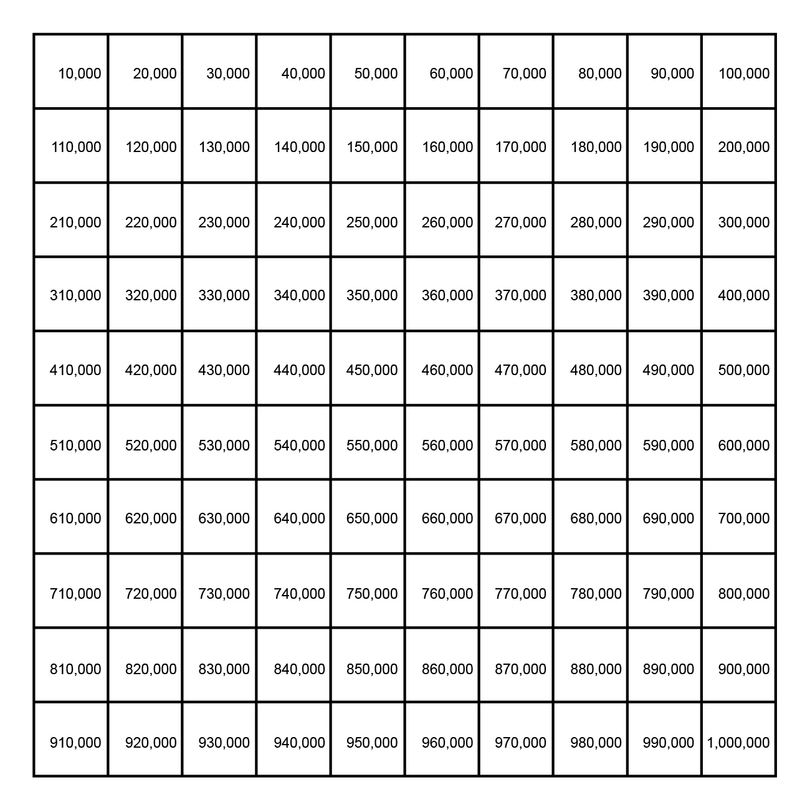Exercise 1.5: Count to one million in hundred thousands and in ten thousands

1. Count aloud from zero to one million in hundred thousands. Then write down the numbers and their names.

0: zero
100,000: one hundred thousand
200,000: two hundred thousand
300,000: three hundred thousand
400,000: four hundred thousand
500,000: five hundred thousand
600,000: six hundred thousand
700,000: seven hundred thousand
800,000: eight hundred thousand
900,000: nine hundred thousand
1,000,000: one million

2. Count aloud from nine hundred thousand to one million in ten thousands. Then write down the numbers and their names.

900,000: nine hundred thousand
910,000: nine hundred and ten thousand
920,000: nine hundred and twenty thousand
930,000: nine hundred and thirty thousand
940,000: nine hundred and forty thousand
950,000: nine hundred and fifty thousand
960,000: nine hundred and sixty thousand
970,000: nine hundred and seventy thousand
980,000: nine hundred and eighty thousand
990,000: nine hundred and ninety thousand
1,000,000: one million

The following counting chart shows you how to count from 990,000 to 1,000,000 in hundreds and in thousands.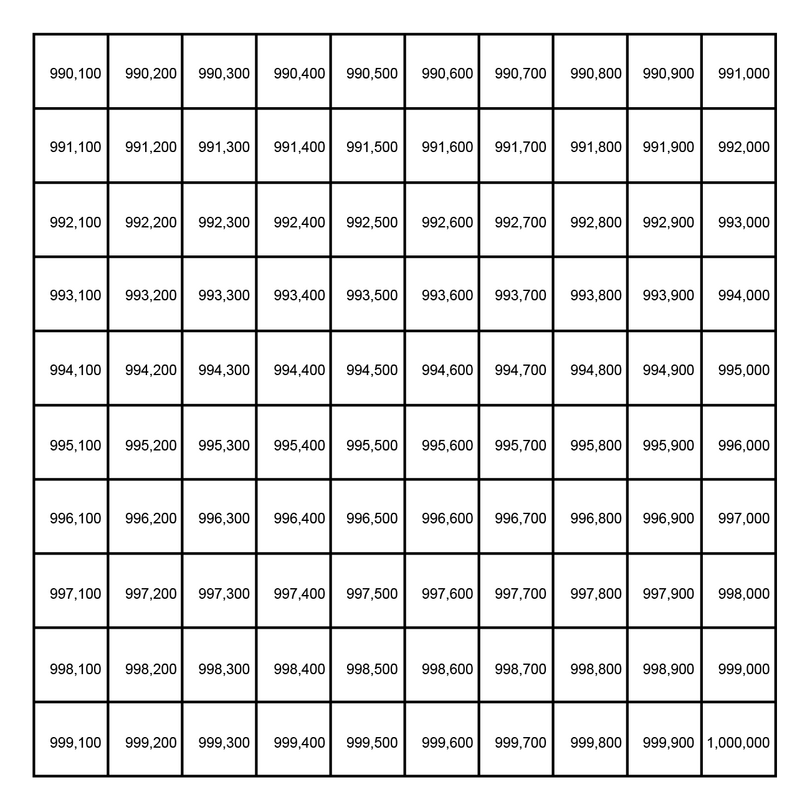Exercise 1.6: Count to one million in thousands and in hundreds

1. Count aloud from nine hundred and ninety thousand to one million in thousands. Then write down the numbers and their names.

990,000: nine hundred and ninety thousand
991,000: nine hundred and ninety one thousand
992,000: nine hundred and ninety two thousand
993,000: nine hundred and ninety three thousand
994,000: nine hundred and ninety four thousand
995,000: nine hundred and ninety five thousand
996,000: nine hundred and ninety six thousand
997,000: nine hundred and ninety seven thousand
998,000: nine hundred and ninety eight thousand
999,000: nine hundred and ninety nine thousand
1,000,000: one million

2. Count aloud from nine hundred and ninety eight thousand five hundred to one million in hundreds. Then write down the numbers and their names.

998,500: nine hundred and ninety eight thousand five hundred
998,600: nine hundred and ninety eight thousand six hundred
998,700: nine hundred and ninety eight thousand seven hundred
998,800: nine hundred and ninety eight thousand eight hundred
998,900: nine hundred and ninety eight thousand nine hundred
999,000: nine hundred and ninety nine thousand
999,100: nine hundred and ninety nine thousand one hundred
999,200: nine hundred and ninety nine thousand two hundred
999,300: nine hundred and ninety nine thousand three hundred
999,400: nine hundred and ninety nine thousand four hundred
999,500: nine hundred and ninety nine thousand five hundred
999,600: nine hundred and ninety nine thousand six hundred
999,700: nine hundred and ninety nine thousand seven hundred
999,800: nine hundred and ninety nine thousand eight hundred
999,900: nine hundred and ninety nine thousand nine hundred
1,000,000: one million

This counting chart shows you how to count from 999,900 to 1,000,000 in units and in tens.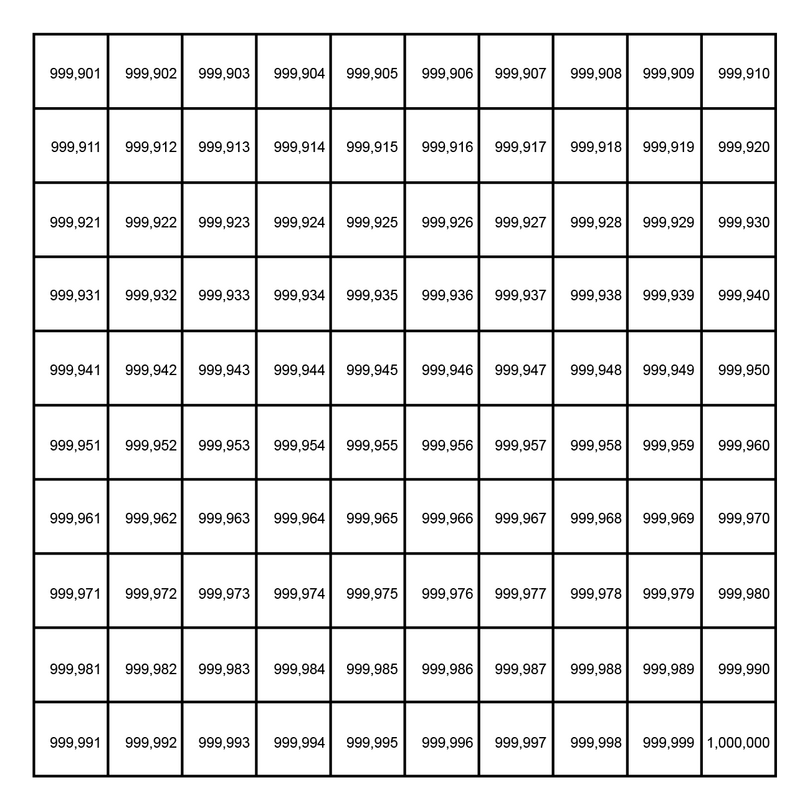Exercise 1.7: Count to one million in tens and in units

1. Count aloud from nine hundred and ninety nine thousand nine hundred to one million in tens. Then write down the numbers and their names.

999,900: nine hundred and ninety nine thousand nine hundred
999,910: nine hundred and ninety nine thousand nine hundred and ten
999,920: nine hundred and ninety nine thousand nine hundred and twenty
999,930: nine hundred and ninety nine thousand nine hundred and thirty
999,940: nine hundred and ninety nine thousand nine hundred and forty
999,950: nine hundred and ninety nine thousand nine hundred and fifty
999,960: nine hundred and ninety nine thousand nine hundred and sixty
999,970: nine hundred and ninety nine thousand nine hundred and seventy
999,980: nine hundred and ninety nine thousand nine hundred and eighty
999,990: nine hundred and ninety nine thousand nine hundred and ninety
1,000,000: one million

2. Count aloud from nine hundred and ninety nine thousand nine hundred and eighty five to one million in units. Then write down the numbers and their names.

999,985: nine hundred and ninety nine thousand nine hundred and eighty five
999,986: nine hundred and ninety nine thousand nine hundred and eighty six
999,987: nine hundred and ninety nine thousand nine hundred and eighty seven
999,988: nine hundred and ninety nine thousand nine hundred and eighty eight
999,989: nine hundred and ninety nine thousand nine hundred and eighty nine
999,990: nine hundred and ninety nine thousand nine hundred and ninety
999,991: nine hundred and ninety nine thousand nine hundred and ninety one
999,992: nine hundred and ninety nine thousand nine hundred and ninety two
999,993: nine hundred and ninety nine thousand nine hundred and ninety three
999,994: nine hundred and ninety nine thousand nine hundred and ninety four
999,995: nine hundred and ninety nine thousand nine hundred and ninety five
999,996: nine hundred and ninety nine thousand nine hundred and ninety six
999,997: nine hundred and ninety nine thousand nine hundred and ninety seven
999,998: nine hundred and ninety nine thousand nine hundred and ninety eight
999,999: nine hundred and ninety nine thousand nine hundred and ninety nine
1,000,000: one million

Counting up to one billion

The table in the previous section listed the names of large numbers made up of groups of ten. This table shows how to write numbers as powers of ten.

The number 1,000,000,000 is named one billion. Try to imagine how large one billion is:

• One billion seconds from your birth, you will be just over 31 years and 8 months old.
• One billion sheets of photocopy paper stacked on top of each other would reach almost 110 km into the sky.

We may count from zero to ten million in millions:
0: zero
1,000,000: one million
2,000,000: two million
3,000,000: three million
4,000,000: four million
5,000,000: five million
6,000,000: six million
7,000,000: seven million
8,000,000: eight million
9,000,000: nine million
10,000,000: ten million

This counting chart may be used to count to 1,000,000,000 in ten millions and in hundred millions.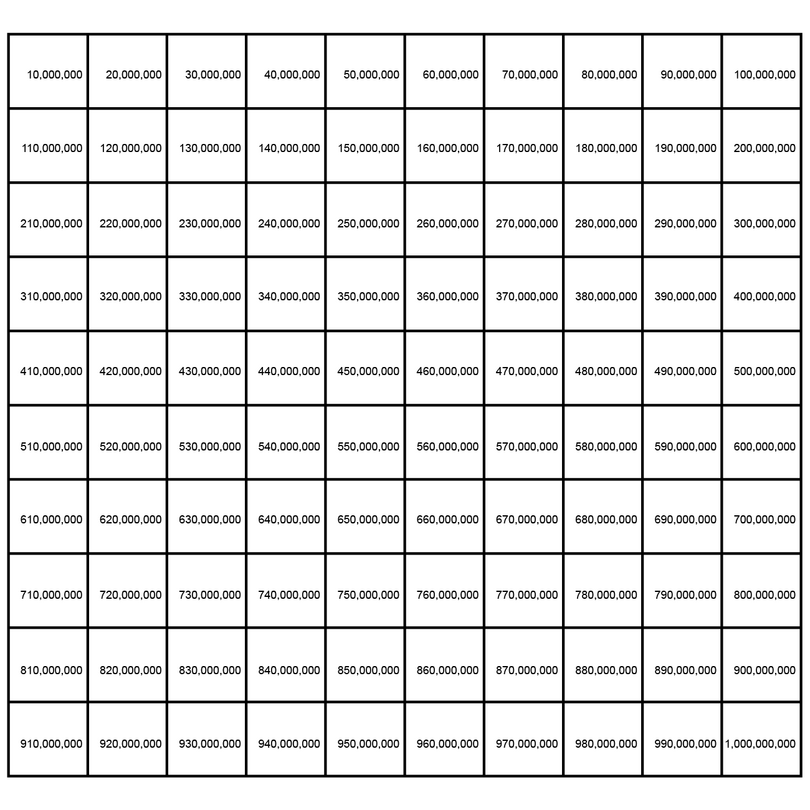This counting chart shows you how to count from 999,990 to 1,000,000,000 in hundreds and in thousands.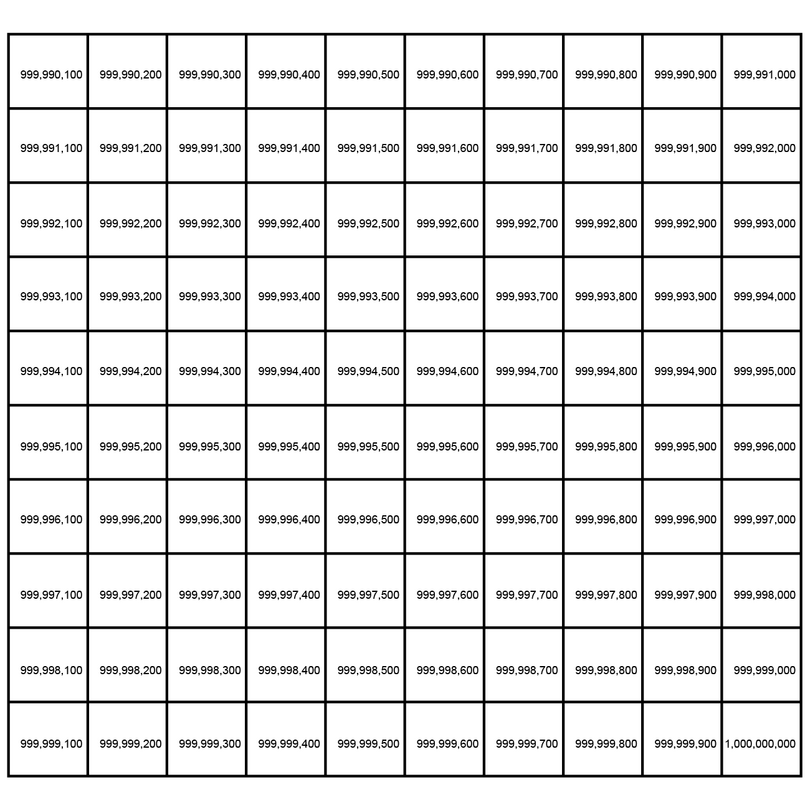Worked example 1.4: Counting with large numbers

Count in thousands from five hundred and sixty one million seven hundred and twenty one thousand to five hundred and sixty one million seven hundred and thirty two thousand. Write down the numbers and their names.

1. Step 1: Write both numbers in a place value table.

2. Step 2: Look closely at the first place value table. Decide in which column the digit must increase as you are counting.

If you count in thousands, the digit in the â€œthâ€ column must increase.

3. Step 3: Increase the digit in the column you identified by 1 and write down the number. Continue until you reach the digit 9.

561,722,000
561,723,000
561,724,000
561,725,000
561,726,000
561,727,000
561,728,000
561,729,000

4. Step 4: Deal with the digit 9 as you did before. Change it to 0 and add 1 to the column to its left. Add the number to your list.

561,722,000
561,723,000
561,724,000
561,725,000
561,726,000
561,727,000
561,728,000
561,729,000
561,730,000

5. Step 5: Carry on increasing the digit in the column you identified in Step 2 by 1, until you reached the required number.

561,722,000
561,723,000
561,724,000
561,725,000
561,726,000
561,727,000
561,728,000
561,729,000
561,730,000
561,731,000
561,732,000

6. Step 6: Compare the last number on your list to the second place value table in Step 1. If they are the same, write down the full number list with names.

561,721,000: five hundred and sixty one million seven hundred and twenty one thousand
561,722,000: five hundred and sixty one million seven hundred and twenty two thousand
561,723,000: five hundred and sixty one million seven hundred and twenty three thousand
561,724,000: five hundred and sixty one million seven hundred and twenty four thousand
561,725,000: five hundred and sixty one million seven hundred and twenty five thousand
561,726,000: five hundred and sixty one million seven hundred and twenty six thousand
561,727,000: five hundred and sixty one million seven hundred and twenty seven thousand
561,728,000: five hundred and sixty one million seven hundred and twenty eight thousand
561,729,000: five hundred and sixty one million seven hundred and twenty nine thousand
561,730,000: five hundred and sixty one million seven hundred and thirty thousand
561,731,000: five hundred and sixty one million seven hundred and thirty one thousand
561,732,000: five hundred and sixty one million seven hundred and thirty two thousand

Exercise 1.8: Count with large numbers

1. Count in millions from two hundred and sixteen million one hundred and fifty four thousand one hundred to two hundred and twenty one million one hundred and fifty four thousand one hundred. Write down the numbers and their names.

If we count in millions, the digit in the â€œmâ€ column must change.

216,154,100: two hundred and sixteen million one hundred and fifty four thousand one hundred
217,154,100: two hundred and seventeen million one hundred and fifty four thousand one hundred
218,154,100: two hundred and eighteen million one hundred and fifty four thousand one hundred
219,154,100: two hundred and nineteen million one hundred and fifty four thousand one hundred
220,154,100: two hundred and twenty million one hundred and fifty four thousand one hundred
221,154,100: two hundred and twenty one million one hundred and fifty four thousand one hundred

2. Count in hundred thousands from nine million eight hundred and ninety six thousand five hundred to ten million three hundred and ninety six thousand five hundred. Write down the numbers and their names.

If we count in hundred thousands, the digit in the â€œhthâ€ column must change.

9,896,500: nine million eight hundred and ninety six thousand five hundred
9,996,500: nine million nine hundred and ninety six thousand five hundred
10,096,500: ten million and ninety six thousand five hundred
10,196,500: ten million one hundred and ninety six thousand five hundred
10,296,500: ten million two hundred and ninety six thousand five hundred
10,396,500: ten million three hundred and ninety six thousand five hundred

3. Count in hundreds from nine hundred and ninety nine million nine hundred and eighty nine thousand seven hundred to nine hundred and ninety nine million nine hundred and ninety thousand two hundred. Write down the numbers and their names.

If we count in hundreds, the digit in the â€œhâ€ column must change.

999,989,700: nine hundred and ninety nine million nine hundred and eighty nine thousand seven hundred
999,989,800: nine hundred and ninety nine million nine hundred and eighty nine thousand eight hundred
999,989,900: nine hundred and ninety nine million nine hundred and eighty nine thousand nine hundred
999,990,000: nine hundred and ninety nine million nine hundred and ninety thousand
999,990,100: nine hundred and ninety nine million nine hundred and ninety thousand one hundred
999,990,200: nine hundred and ninety nine million nine hundred and ninety thousand two hundred

Counting in millions and billions

In everyday life, we commonly use a mixture of digits and words to express (read and write) large numbers. For example, most people will find it easier to read 25 billion than 25,000,000,000.

To help us interpret a mixture of digits and words, we can think about what they mean. We can think of 25 billion as 25 groups of 1,000,000,000. This is the same as 25Â Ã—Â 1,000,000,000. Therefore, 25 billion is equal to 25,000,000,000.

Worked example 1.5: Writing a mixture of digits and words in digits only

Express 0.15 billion in digits only. Write out the full name of the answer.

1. Step 1: Write down the meaning of the mixed number.

0.15 billion means 0.15 of a group of 1,000,000,000:
$0.15\times1,000,000,000$

2. Step 2: Do the calculation.

• â€œIgnoreâ€ the decimal point at first.
$15\times1,000,000,000=15,000,000,000$
• Insert a decimal point in your answer. You must have the same number of digits after the decimal point as there are altogether in the original numbers: 1,000,000,000 does not have a decimal point and 0.15 has two digits after the decimal point, so you need two digits after the decimal point in your answer.
$15,000,000,000$ becomes $15,000,000,0.00$
• If there are zeros at the end of the number after the decimal point, you can leave them out. Rewrite the number and regroup it correctly. $150000000=150,000,000$
3. Step 3: Write the full name of the answer. Use the grouping commas to guide you.

• The numbers from 0 to 999 have no grouping commas.
• The numbers from 1,000 to 999,999 have only one grouping comma.
• The numbers from 1,000,000 to 999,999,999 have two grouping commas.
• The numbers from 1,000,000,000 to 999,999,999,999 have three grouping commas.

150,000,000 has two grouping commas, so it is in the millions. Its full name is: one hundred and fifty million.

Exercise 1.9: Express a mixture of digits and words in digits only

1. Express 0.835 million in digits only, and write out its full name.

Without the decimal point: $835\times1,000,000=835,000,000$
With the decimal point: 835000.000
Name: Eight hundred and thirty five thousand

2. Express 3.45 billion in digits only, and write out its full name.

Without the decimal point: $345\times1,000,000,000=345,000,000,000$
With the decimal point: 3450000000.00
Name: Three billion four hundred and fifty million

3. Express 20.05 million in digits only, and write out its full name.

Without the decimal point: $2,005\times1,000,000=2,005,000,000$
With the decimal point: 20050000.00
Name: Twenty million and fifty thousand

4. Express 175.5 million in digits only, and write out its full name.

Without the decimal point: $1,755\times1,000,000=1,755,000,000$
With the decimal point: 175500000.0
Name: One hundred and seventy five million five hundred thousand

5. Express 202.25 million in digits only, and write out its full name.

Without the decimal point: $20,225\times1,000,000=20,225,000,000$
With the decimal point: 202250000.00
Name: Two hundred and two million two hundred and fifty thousand

Exercise 1.10: Count in millions and billions

1. Count in millions from 1.5 million to 5.5 million. Write down the numbers as digits only and include their full names.

1.5 million = 1,500,000: one million five hundred thousand
2.5 million = 2,500,000: two million five hundred thousand
3.5 million = 3,500,000: three million five hundred thousand
4.5 million = 4,500,000: four million five hundred thousand
5.5 million = 5,500,000: five million five hundred thousand

2. Count in 0.5 billions from 10.5 billion to 12 billion. Write down the numbers as digits only and include their full names.

10.5 billion = 10,500,000,000: ten billion five hundred million
11 billion = 11,000,000,000: eleven billion
11.5 billion = 11,500,000,000: eleven billion five hundred million
12 billion = 12,000,000,000: twelve billion

3. Count in 0.25 billions from 25 billion to 26.5 billion. Write down the numbers as digits only and include their full names.

25 billion = 25,000,000,000: twenty five billion
25.25 billion = 25,250,000,000: twenty five billion two hundred and fifty million
25.5 billion = 25,500,000,000: twenty five billion five hundred million
25.75 billion = 25,750,000,000: twenty five billion seven hundred and fifty million
26 billion = 26,000,000,000: twenty six billion
26.25 billion = 26,250,000,000: twenty six billion two hundred and fifty million
26.5 billion = 26,500,000,000: twenty six billion five hundred million

4. Count in 3 millions from 900 million to 912 million. Write down the numbers as digits only and include their full names.

900 million = 900,000,000: nine hundred million
903 million = 903,000,000: nine hundred and three million
906 million = 906,000,000: nine hundred and six million
909 million = 909,000,000: nine hundred and nine million
912 million = 912,000,000: nine hundred and twelve million

5. Count in 2 millions from 990 million to 1,000 million. Write down the numbers as digits only and include their full names.

990 million = 990,000,000: nine hundred and ninety million
992 million = 992,000,000: nine hundred and ninety two million
994 million = 994,000,000: nine hundred and ninety four million
996 million = 996,000,000: nine hundred and ninety six million
998 million = 998,000,000: nine hundred and ninety eight million
1,000 million = 1,000,000,000: one billion

Counting in trillions

Our table with powers of ten can be expanded further, to include even larger numbers.

The number 1,000,000,000,000 is named one trillion. Try to imagine how large one trillion is:

• A human cannot live for one trillion seconds; it is equal to almost 31,708 years.
• Suppose the whole surface of Nigeria is tiled with 50 kobo coins. One trillion 50 kobo coins will form 412 layers over the surface of Nigeria.

Worked example 1.6: Comparing large numbers

Arrange the following numbers from the smallest to the largest:
50.5 billion; 0.055 trillion; 500.5 million

1. Step 1: Write all the numbers as digits only.

50.5 billion without the decimal point: $505\times1,000,000,000=505,000,000,000$
With the decimal point: 50500000000.0
Correctly grouped: 50,500,000,000

0.055 trillion without the decimal point: $0.055\times1,000,000,000,000=55,000,000,000,000$
With the decimal point: 55000000000.000
Correctly grouped: 55,000,000,000

500.5 million without the decimal point: $5,005\times1,000,000=5,005,000,000$
With the decimal point: 500500000.0
Correctly grouped: 500,500,000

2. Step 2: Write the numbers one below the other. Align them on the last digit.

3. Step 3: Use the list from Step 2 to write the numbers in the required order.

From Step 2 is is easy to compare the numbers. Arranged from small to large:

Therefore, the numbers arranged from smallest to largest are 500.5 million; 50.5 billion; 0.055 trillion.

Exercise 1.11: Compare large numbers

1. Which number is larger, 0.08 trillion or 800 billion?

0.08 trillion without the decimal point: $8\times1,000,000,000,000=8,000,000,000,000$
With the decimal point: 80000000000.00
Correctly grouped: 80,000,000,000
This is 80 billion.

Therefore, 800 billion is the larger number.

2. Which number is smaller, 120 billion or 1.2 trillion?

1.2 trillion without the decimal point: $12\times1,000,000,000,000=12,000,000,000,000$
With the decimal point: 1200000000000.0
Correctly grouped: 1,200,000,000,000

120 billion: $120\times1,000,000,000=120,000,000,000$

Compare the numbers:
$\begin{array}{r} 1,200,000,000,000 \newline 120,000,000,000 \newline \end{array}$

Therefore, 120 billion is the smaller number.

3. Arrange the following numbers from the largest to the smallest: 3,600 million; 3 billion; 0.36 trillion

3,600 million: $3,600\times1,000,000=3,600,000,000$

3 billion: $3\times1,000,000,000=3,000,000,000$

0.36 trillion without the decimal point: $36\times1,000,000,000,000=36,000,000,000,000$
With the decimal point: 360000000000.00
Correctly grouped: 360,000,000,000

Compare the numbers:
$\begin{array}{r} 3,600,000,000 \newline 3,000,000,000 \newline 360,000,000,000 \newline \end{array}$

Therefore, the numbers from the largest to the smallest are 0.36 trillion; 3,600 million; 3 billion.

Exercise 1.12: Count in trillions

1. Count in trillions from 3.5 trillion to 6.5 trillion. Write down the numbers as digits only and include their full names.

3.5 trillion = 3,500,000,000,000: three trillion five hundred billion
4.5 trillion = 4,500,000,000,000: four trillion five hundred billion
5.5 trillion = 5,500,000,000,000: five trillion five hundred billion
6.5 trillion = 6,500,000,000,000: six trillion five hundred billion

2. Count in 0.5 trillions from 37.5 trillion to 40.5 trillion. Write down the numbers as digits only and include their full names.

37.5 trillion = 37,500,000,000,000: thirty seven trillion five hundred billion
38 trillion = 38,000,000,000,000: thirty eight trillion
38.5 trillion = 38,500,000,000,000: thirty eight trillion five hundred billion
39 trillion = 39,000,000,000,000: thirty nine trillion
39.5 trillion = 39,500,000,000,000: thirty nine trillion five hundred billion
40 trillion = 40,000,000,000,000: forty trillion
40.5 trillion = 40,500,000,000,000: forty trillion five hundred billion

3. Count in 0.25 trillions from 9 trillion to 10.5 trillion. Write down the numbers as digits only and include their full names.

9 trillion = 9,000,000,000,000: nine trillion
9.25 trillion = 9,250,000,000,000: nine trillion two hundred and fifty billion
9.5 trillion = 9,500,000,000,000: nine trillion five hundred billion
9.75 trillion = 9,750,000,000,000: nine trillion seven hundred and fifty billion
10 trillion = 10,000,000,000,000: ten trillion
10.25 trillion = 10,250,000,000,000: ten trillion two hundred and fifty billion
10.5 trillion = 10,500,000,000,000: ten trillion five hundred billion

4. Count in 3 trillions from 987 trillion to 999 trillion. Write down the numbers as digits only and include their full names.

987 trillion = 987,000,000,000,000: nine hundred and eighty seven trillion
990 trillion = 990,000,000,000,000: nine hundred and ninety trillion
993 trillion = 993,000,000,000,000: nine hundred and ninety three trillion
996 trillion = 996,000,000,000,000: nine hundred and ninety six trillion
999 trillion = 999,000,000,000,000: nine hundred and ninety nine trillion

5. Count in 4 trillions from 892 trillion to 908 trillion. Write down the numbers as digits only and include their full names.

892 trillion = 892,000,000,000,000: eight hundred and ninety two trillion
896 trillion = 896,000,000,000,000: eight hundred and ninety six trillion
900 trillion = 900,000,000,000,000: nine hundred trillion
904 trillion = 904,000,000,000,000: nine hundred and four trillion
908 trillion = 908,000,000,000,000: nine hundred and eight trillion

1.6 Using large numbers in everyday life

In your daily life, you may come across large numbers in newspapers, on television shows and in some of your other school subjects.

Whenever you get stuck with a large number, write the number in a place value table to help you make sense of it. Remember the order of the place value table:

Exercise 1.13: Use large numbers to solve problems

1. Scientists estimate that the average child has $10^5$ hairs on her head.

1. Write out the full number in digits.
1. Give the name of this number.

One hundred thousand

2. During May 2019, Nigeriaâ€™s population was recorded as 200,233,151.

1. Write the number in words.

Two hundred million two hundred and thirty three thousand one hundred and fifty one

1. It is estimated that the population will almost double by 2050 to 400,400,000. Write this as a mixture of digits and words, so that it will be easier to read.

Use a place value table to help you.
$% <![CDATA[ \begin{array}{|c|c|c|c|c|c|c|c|c|c|c|c|c|} \hline \text{trillion} &\text{hb} &\text{tb} &\text{billion} & \text{hm} & \text{tm} & \text{million} &\text{hth} & \text{tth} & \text{thousand} & \text{ h } & \text{ t } & \text{ u } \newline \hline & & & & 4 & 0 & 0 & 4 & 0 & 0 & 0 & 0 & 0 \newline \hline \end{array} %]]>$
The number can be written as 400.4 million.

3. In 2018, the Federal Government of Nigeria estimated a total revenue of â‚¦6,607,000,000,000.

1. Write the number as a mixture of digits and words, so that it will be easier for the general public to read.

Use a place value table to help you.
$% <![CDATA[ \begin{array}{|c|c|c|c|c|c|c|c|c|c|c|c|c|} \hline \text{trillion} &\text{hb} &\text{tb} &\text{billion} & \text{hm} & \text{tm} & \text{million} &\text{hth} & \text{tth} & \text{thousand} & \text{ h } & \text{ t } & \text{ u } \newline \hline 6 & 6 & 0 & 7 & 0 & 0 & 0 & 0 & 0 & 0 & 0 & 0 & 0 \newline \hline \end{array} %]]>$
The number can be written as â‚¦6.607 trillion.

1. It was estimated that oil revenue would be â‚¦2.442 trillion of the total revenue and non-oil revenue would be â‚¦4,165,000,000,000. Determine which revenue is more, the oil revenue or the non-oil revenue.

$2.442\times1,000,000,000,000=2,442,000,000,000$
$% <![CDATA[ \begin{array}{l r} \text{Oil:} & â‚¦2,442,000,000,000 \newline \text{Non-oil:} & â‚¦4,165,000,000,000 \newline \end{array} %]]>$
The non-oil revenue is more.

4. The side length of a cube is 1 m.

1. Convert the side length to centimetres. Then determine the volume of the cube in cubic centimetres. Write the answer in words.

1 m = 100 cm
$% <![CDATA[ \begin{array}{l l} \text{volume} &=\text{side}\times\text{side}\times\text{side} \newline &=100\times100\times100\newline &=1,000,000\text{ cm}^3 \end{array} %]]>$
The volume is one million cubic centimetres.

1. Convert the side length to millimetres. Then determine the volume of the cube in cubic millimetres. Write the answer in words.

1 m = 1,000 mm
$% <![CDATA[ \begin{array}{l l} \text{volume} &=\text{side}\times\text{side}\times\text{side} \newline &=1,000\times1,000\times1,000\newline &=1,000,000,000\text{ mm}^3 \end{array} %]]>$
The volume is one billion cubic millimetres.

5. There are 31,536,000 seconds in one year.

1. Calculate the number of seconds in ten years. Write the answer in words.

$31,536,000\times10=315,360,000$
There are three hundred and fifteen million three hundred and sixty thousand seconds in ten years.

1. Determine whether your previous answer is more or less than 0.3 billion.

$0.3\times1,000,000,000=300,000,000$
The previous answer is more than 0.3 billion.

6. In 2018, the Federal Government of Nigeria estimated the following budget allocations to three of their ministries: â‚¦435.01 billion to Education, â‚¦444.43 billion to Defence and â‚¦269.34 billion to Health. Calculate the total allocation to all three ministries. Write the full number and give its name.

$% <![CDATA[ \begin{array}{r r r} & 435.01\times1,000,000,000 & =\space435,010,000,000 \newline +& 444.43\times1,000,000,000 & =\space444,430,000,000 \newline +& 269.34\times1,000,000,000 & =\space269,340,000,000 \newline \hline & & \space \space 1,148,780,000,000\newline \hline \end{array} %]]>$
The total allocation is â‚¦1,148,780,000,000. It is one trillion one hundred and forty eight billion seven hundred and eighty million naira.

1.7 Summary

• In the base ten number system, we use the digits 0; 1; 2; 3; 4; 5; 6; 7; 8; 9 to represent any other number.
• The numbers 0; 1; 2; 3; 4; 5; 6; 7; 8; 9 are called units in the base ten number system.
• The numbers 10; 20; 30; 40; 50; 60; 70; 80; 90 are called tens in the base ten number system.
• The numbers 100; 200; 300; 400; 500; 600; 700; 800; 900 are called hundreds in the base ten number system.
• The numbers 1,000; 2,000; 3,000; 4,000; 5,000; 6,000; 7,000; 8,000; 9,000 are called thousands in the base ten number system.
• The numbers 10,000; 20,000; 30,000; 40,000; 50,000; 60,000; 70,000; 80,000; 90,000 are called ten thousands in the base ten number system.
• Decimal fractions are written using the base ten number system. The digits of the numerator are written after a decimal point. The denominator is 10; 100; 1,000; 10,000 and so on. For example, the decimal fraction 0.25 is equal to $\frac{25}{100}$.
• We use commas to group the digits in large numbers in threes, so that they are easier to read. We group the digits from the decimal point, going to the left. For example, 4589762.35 is written as 4,589,762.35.
• The place value of a digit is the value of that digit according to its position in the number.
• We may use powers of ten to express large numbers. The power tells us how many times 10 must be multiplied by itself. For example, $10^4$ means $10\times10\times10\times10$. Here is a list of the powers of ten. $% <![CDATA[ \begin{array}{|l|l|l|} \hline \textbf{Name} & \textbf{Number} & \textbf{Powers of ten} \newline \hline \text{Ten} & 10 & 10=10^1 \newline \hline \text{Hundred} & 100 & 10\times10=10^2 \newline \hline \text{Thousand} & 1,000 & 10\times10\times10=10^3 \newline \hline \text{Ten thousand} & 10,000 & 10\times10\times10\times10=10^4 \newline \hline \text{Hundred thousand} & 100,000 & 10\times10\times10\times10\times10=10^5 \newline \hline \text{Million} & 1,000,000 & 10\times10\times10\times10\times10\times10=10^6 \newline \hline \text{Ten million} & 10,000,000 & 10\times10\times10\times10\times10\times10\times10=10^7 \newline \hline \text{Hundred million} & 100,000,000 & 10\times10\times10\times10\times10\times10\times10\times10=10^8 \newline \hline \text{Billion} & 1,000,000,000 & 10\times10\times10\times10\times10\times10\times10\times10\times10=10^9 \newline \hline \text{Ten billion} & 10,000,000,000 & 10\times10\times10\times10\times10\times10\times10\times10\times10\times10=10^{10} \newline \hline \text{Hundred billion} & 100,000,000,000 & 10\times10\times10\times10\times10\times10\times10\times10\times10\times10\times10=10^{11} \newline \hline \text{Trillion} & 1,000,000,000,000 & 10\times10\times10\times10\times10\times10\times10\times10\times10\times10\times10\times10=10^{12} \newline \hline \end{array} %]]>$
• We can use the above list to draw up a place value table. This makes it easer to work with large numbers. $% <![CDATA[ \begin{array}{|c|c|c|c|c|c|c|c|c|c|c|c|c|} \hline \text{trillion} &\text{hb} &\text{tb} &\text{billion} & \text{hm} & \text{tm} & \text{million} &\text{hth} & \text{tth} & \text{thousand} & \text{ h } & \text{ t } & \text{ u } \newline \hline & & & & & & & & & & & & \newline \hline \end{array} %]]>$
• We may use a place value table to help us count with large numbers. Whenever we want to proceed after we reached the digit 9 in any column of the table, the 9 becomes 0 and the digit in the column to the left increases by 1.
• Large numbers are sometimes expressed as a mixture of digits and words to make them easier to read. For example, 0.5 billion is the number 500,000,000 with the name five hundred million.